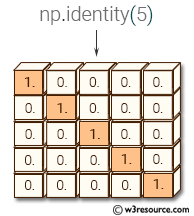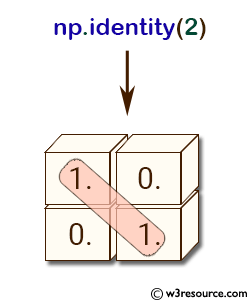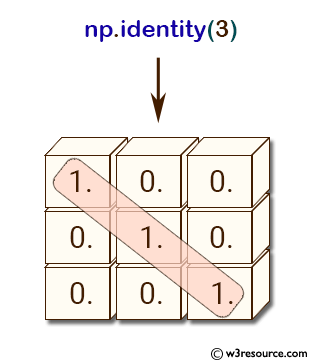# NumPy: numpy.identity() function

## numpy.identity() function

An identity matrix is a square matrix in which all the elements of the main diagonal are equal to 1 and all other elements are equal to 0. The identity() function return the identity array.
This function is useful in linear algebra, where the identity matrix plays an important role in representing the identity transformation in vector spaces. Identity matrices are also useful in testing matrix operations and algorithms, as they have special properties that make them easy to work with.

Syntax:

`numpy.identity(n, dtype=None)`Parameters:

Name Description Required /
Optional
n Number of rows (and columns) in n x n output. Required
dtype Data-type of the output. Defaults to float. optional

Return value:

[ndarray] n x n array with its main diagonal set to one, and all other elements 0.

Example-1: Create an Identity Matrix using NumPy's identity()

``````>>> import numpy as np
>>> np.identity(2)
array([[ 1.,  0.],
[ 0.,  1.]])
``````

In the above example, numpy.identity(2) returns a 2x2 identity matrix with 1s in diagonal and 0s in all other positions.

Pictorial Presentation:Example-2: Create an Identity Matrix using NumPy's identity()

``````>> import numpy as np
>>> np.identity(3, dtype=int)
array([[1, 0, 0],
[0, 1, 0],
[0, 0, 1]])
>>> np.identity(3) #default data type is float
array([[ 1.,  0.,  0.],
[ 0.,  1.,  0.],
[ 0.,  0.,  1.]])
``````

The identity() function in NumPy returns an identity matrix of the specified size. An identity matrix is a square matrix with ones on the diagonal and zeros elsewhere.

In the first example, np.identity(3, dtype=int), the identity matrix of size 3x3 is created with data type 'int'. Therefore, the elements of the matrix are integers.

In the second example, np.identity(3), the identity matrix of size 3x3 is created with default data type 'float'. Therefore, the elements of the matrix are floating-point numbers.

Pictorial Presentation:Example: Create a complex-valued identity matrix with a non-default diagonal

Code:

``````import numpy as np

# Create a 3x3 complex-valued identity matrix with diagonal elements of 1+1j
arr = np.identity(3, dtype=complex, k=1) * (1 + 1j)
print(arr)

``````

Output:

```[[0.+0.j 1.+1.j 0.+0.j]
[0.+0.j 0.+0.j 1.+1.j]
[0.+0.j 0.+0.j 0.+0.j]]
```

In the above example, we create a complex-valued identity matrix with dimensions (3, 3) using np.identity() function. We specify the data type as complex and the diagonal offset as 1. We then multiply the resulting matrix with the complex scalar (1+1j), resulting in a matrix with diagonal elements of 1+1j on the first upper diagonal and 0's elsewhere.

Python - NumPy Code Editor:

Previous: eye()
Next: ones()

﻿